Next: Fission barriers for the Up: Theoretical framework and results Previous: Description of pairing correlations

## Comparison of pairing models

Figures 1, 2, and 3 display total binding energies (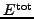), mass hexadecapole moments (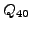), and neutron/proton pairing gaps (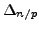) calculated along the static fission paths of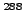Rf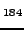,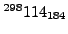, and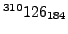. The fission paths were computed with a quadratic constraint on the mass quadrupole moment (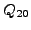). Our study covers the oblate/prolate deformations of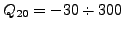b with a step of 10b.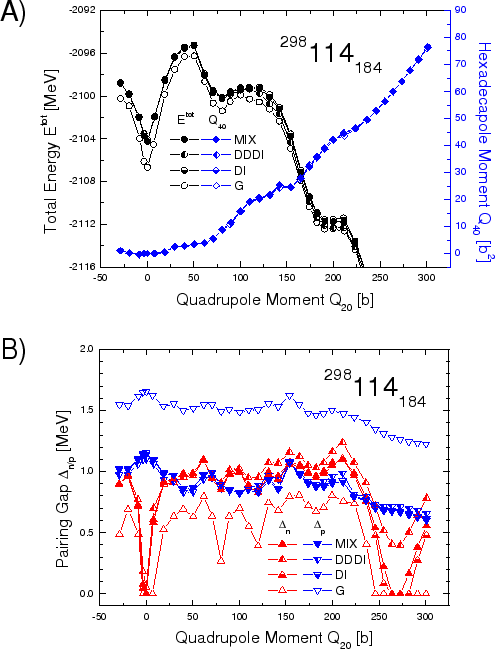As in previous studies based on the SLy4 functional,[21,22] we found that all nuclei considered in this work have spherical shapes in their ground states. This is because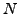=184 appears to be the magic neutron number in most theoretical models based on the Skyrme approach. Due to the magic character of=184 isotones, ground-state neutron pairing gaps calculated in BCS vanish. The ground-state proton pairing gaps, on the other hand, show considerable variations with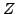. They are large in the open-shell rutherfordium (=104), but in=114 the values of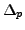are considerably reduced. As expected, proton pairing is much weakened for=126 which is predicted to be semi-magic.[21,22,23]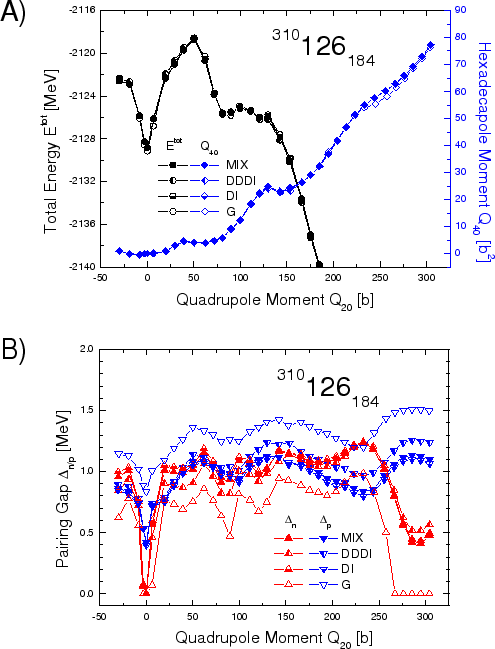The calculated static fission paths have reflection-symmetric shapes, i.e.,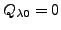for all odd multipolarities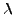. Furthermore, one can see that irrespective of the pairing interaction used, the hexadecapole moments are almost identical, and they gradually increase (from 0 up to about 80b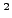) along the calculated static fission paths. In contrast to, the collective potentials (i.e., total energiesas functions of) differ depending on the pairing model employed. This is particularly evident forRf(Fig. 1A) where the fission barrier calculated within the SHF+BCS(G) model is significantly higher as compared to those obtained in the SHF+BCS(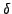) variants. In the case of(Fig. 2A), the fission barriers calculated with the SHF+BCS(G) and SHF+BCS() interactions are considerably closer to one another than in the case ofRf. Furthermore, for(Fig. 3A), all fission barriers calculated with both pairing models are almost identical. This can be attributed to the behavior of proton pairing along the fission paths. Indeed, inRfthere is a large systematic difference betweenvalues in SHF+BCS(G) and SHF+BCS() variants, with the seniority-pairing model producing considerably larger pairing gaps. This difference decreases when going towardsin whichobtained within the SHF+BCS(G) model are much closer to those obtained within the SHF+BCS() model. This result indicates that the isospin dependence of seniority pairing strengths given by Eq. (1) is too strong and thus unrealistic. Another interesting observation is that neutron/proton pairing gaps (hence, corresponding potential energies) calculated within the SHF+BCS() framework are very similar, regardless of the parameterization variant used (MIX, DDDI or DI), see Eq. (4).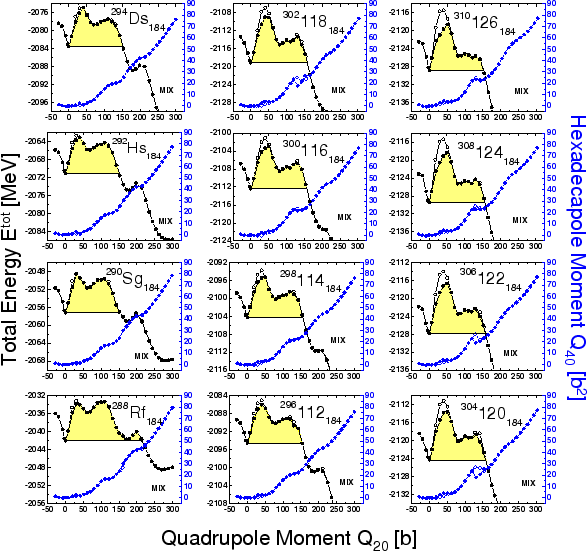Next: Fission barriers for the Up: Theoretical framework and results Previous: Description of pairing correlations
Jacek Dobaczewski 2006-12-10